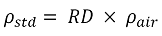# Standard Density Calculation (based on Relative Density)

### Standard Density Calculation (based on Relative Density)

#### This method is used to calculate the standard density from the relative density

Standard density is calculated as follows:Symbol Description Units
ρstd Standard density kg/Sm3
RD Relative density -

Constant Description Value Units
ρair Standard density of dry air 1.225410 kg/Sm3